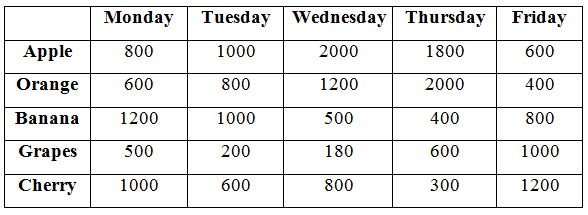# SBI PO Prelims Quantitative Aptitude Questions 2019 – Day 06

SBI PO 2019 Notification will be expected soon. It is one of the most expected recruitment among the banking aspirants. Every year the exam pattern for SBI PO has been changing. Depends upon the changing of exam pattern the questions are quite harder compare to the previous year. So the questions are in high level than the candidate’s assumption.

As per the latest trend, our IBPS Guide is providing the updated New Exam Pattern Quantitative Aptitude questions for SBI PO 2019 Day 6. Our Skilled experts were mounting the questions based on the aspirant’s needs. So candidates shall start your preparation and practice on daily basis with our SBI PO pattern quantitative aptitude questions 2019 day 6. Start your effective preparation from the right beginning to get success in upcoming SBI PO 2019.

[WpProQuiz 5042]

### Click Here for SBI PO Pre 2019 High-Quality Mocks Exactly on SBI Standard

Direction (1- 5): Find the wrong term in the following number series?

1) 13440, 1680, 240, 50, 8, 2

a) 8

b) 2

c) 50

d) 240

e) None of these

2) 3, 20, 137, 955, 6671, 46688

a) 137

b) 6671

c) 20

d) 955

e) None of these

3) 4, 16, 112, 450, 7168, 157696

a) 7168

b) 450

c) 112

d) 157696

e) None of these

4) 7, 28, 42, 53, 59, 62

a) 42

b) 59

c) 62

d) 53

e) None of these

5) 19, 25, 55, 169, 679, 3398

a) 55

b) 3398

c) 679

d) 25

e) None of these

Direction (6 – 10): Study the following information carefully and answer the given questions:

The given table shows the production (in kg) of Apple, Orange, Banana, Grapes and Cherry from Monday to Friday in the same week.6) Quantity I: The average production of Cherry in all the 5 days in the week?

Quantity II: The average production of Banana in all the 5 days in the week?

a) Quantity I > Quantity II

b) Quantity I ≥ Quantity II

c) Quantity II > Quantity I

d) Quantity II ≥ Quantity I

e) Quantity I = Quantity II or Relation cannot be established

7) If the price of production of Apple from Monday to Wednesday is

Rs. 180 per kg, Rs. 200 per kg and Rs. 120 per kg respectively and the price of the production of Orange from Wednesday to Friday is Rs. 200 per kg, Rs. 150 per kg and Rs. 120 per kg respectively. The total production cost of Apple from Monday to Wednesday is approximately what percent of the total production cost of Orange from Wednesday to Friday?

a) 82%

b) 75%

c) 99%

d) 113%

e) 66%

8) If the 20%, 10%, 25%, 5% and 15% of the production of grapes from Monday to Friday respectively are damaged, the remaining good grapes are sold at Rs. 40 per kg, Rs. 25 per kg, Rs. 20 per kg, Rs. 30 per kg and Rs. 50 per kg from Monday to Friday respectively. Find the total costs received by selling the grapes from Monday to Friday?

a) Rs.82800

b) Rs.78400

c) Rs.81900

d) Rs.78200

e) None of these

9) Find the difference between the total production of Apple, Orange and Cherry from Monday, Wednesday and Friday respectively and that of the total production of Grapes, Banana and Cherry from Tuesday, Monday and Thursday respectively?

a) 1500

b) 1200

c) 1800

d) 1400

e) None of these

10) If the price of the production of Banana from Wednesday and Friday is Rs.30 per kg and Rs.40 per kg respectively. The total production cost of Banana from Wednesday is approximately what percent of the total production cost of Banana from Friday?

a) 55 %

b) 47 %

c) 38 %

d) 22 %

e) 63 %

Direction (1-5) :

13440 ÷ 8 = 1680

1680 ÷ 7 = 240

240 ÷ 6 = 40

40 ÷ 5 = 8

8 ÷ 4 = 2

The wrong term is, 50

3*7 – 1 = 20

20*7 – 3 = 137

137*7 – 5 = 954

954*7 – 7 = 6671

6671*7 – 9 = 46688

The wrong term is, 955

4*(0+4) = 16

16*(1+6) = 112

112*(1+1+2) = 448

448*(4+4+8) = 7168

7168*(7+1+6+8) = 157696

7 + (3.5*6) = 28

28 + (3*5) = 43

43 + (2.5*4) = 53

53 + (2*3) = 59

59 + (1.5*2) = 62

The wrong term is, 42

19*1 + 6 = 25

25*2 + 5 = 55

55*3 + 4 = 169

169*4 + 3 = 679

679*5 + 2 = 3397

The wrong term is, 3397

Direction (6-10) :

From Quantity I,

Average = (1000+600+800+300+1200)/5 = 3900/5 = 780

From Quantity II,

Average = (1200+1000+500+400+800)/5 = 3900/5 = 780

Total production cost of Apple from Monday to Wednesday

= > (180*800 + 200*1000 + 120*2000) = 584000

Total production cost of Orange from Wednesday to Friday

= > (200*1200 + 150*2000 + 120*400) = 588000

Required percentage = (584000/588000)*100 = 99%

Grapes = 500*(80/100)*40 + 200*(90/100)*25 + 180*(75/100)*20 + 600*(95/100)*30 + 1000*(85/100)*50

= > 16000 + 4500 + 2700 + 17100 + 42500 = Rs. 82800

Required difference = (800+1200+1200) – (200+1200+300)

= > 3200 – 1700 = 1500

Production cost of Banana from Wednesday = 500*30 = 15000

Production cost of Banana from Friday = 40*800 = 32000

Required percentage = (15000/32000)*100 = 46.8% = 47 %

### Click Here for SBI PO Pre 2019 High-Quality Mocks Exactly on SBI Standard